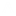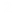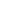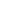```
1 {-
2
3 Pearl 1: Smallest Free Number
4
5 Problem:
6 Assume X is a list of distinct Natural numbers (non-negative integers), in no particular order.
7 Find the smallest number not in the list.
8
9 We will show two algorithms that do it in LINEAR time.
10 On first sight, it is not clear such exist. After all, sorting a list is no linear time.
11 But there's a trick.
12
13 The key trick: not every number in the range [0..length xs] can be in xs!
14 Thus, the smallest number NOT in xs is then the smallest number not in
15 filter (<=n) xs, where n=length xs.
16
17 The two programs:
18 Array based: builds a checklist of the numbers present in filter (<=n) xs.
19
20 Divide and conquer:
21 express minfree(xs++ys) in terms of minfree xs  and minfree ys.
22
23 -}
24 module P1 where
25
26 import Data.Array     (Array, elems, accumArray)
27 import Data.List     (partition)
28 testX = [8,23,9,0,12,11,1,10,13,7,41,21,5,17,3,19,2,6] :: [Int]
29
30 type Nat = Int     -- Just for ease of reading things here. Not correct!!
31
32 -- Brute force approach
33 -- Requires O(N^2) steps
34 -- (see implemntation of (\\) )
35 minfree :: [Nat] -> Nat
36 minfree xs = head ([0..] \\ xs)
37
38 -- where  us (\\) vs    denotes the list of those elements in us remaining after removing
39 -- any elements in vs.
40 (\\)    :: Eq a => [a] -> [a] -> [a]
41 us \\ vs = filter ( `notElem` vs) us
42
43 -- Array based method: Build the checklist as an array
44 -- We will use the 'tricky' function accumArray, which will enable us to do it in linear time.
45 -- accumArray: Constructs an immutable array from a list of associations.
46 -- Unlike array, the same index is allowed to occur multiple times in the list of associations;
47 -- an accumulating function is used to combine the values of elements with the same index.
48
49 checkList         :: [Nat] -> Array Nat Bool
50 checkList xs     =  accumArray         --
51                     (||)             -- accumlating function. not realy used, as no duplicates!
52                     False            -- inital entry for each index
53                     (0,n)            -- (lower/upper) indices of new array
54                     (zip (filter (<= n) xs) (repeat True) )    -- index-value pairs
55                     where
56                         n = length xs
57 -- Just for fun: Another use of accumArray: Sorting array in LINEAR time, if we know all
58 -- values lie between (0,n)
59 -- in our case, we can then look for the first '0' in the resulting array
60
61 countList        ::  [Nat] -> Array Nat Nat
62 countList xs    =   accumArray (+) 0 (0,n) ( zip xs (repeat 1))
63                     where
64                         n = length xs
65
66 -- Once we have the array, finding the minimum is simple:
67 -- (elems converts array into a list)
68 search :: Array Nat Bool -> Nat
69 search = length.takeWhile id.elems
70
71 -- Divide and conquer
72 minFree xs            =    minFrom 0 (length xs,xs)
73 minFrom a (n,xs)    |    n==0        = a
74                     |    m==b-a        = minFrom b (n-m,vs)    -- if us has exactly the number of spots,
75                                                             -- and since there's no repetiion, it means
76                                                             -- the gap have to be in the OTHER series:
77                                                             -- so we continue the search with vs
78                     |    otherwise    = minFrom a (m,us)        -- if us had the gap ==> keep searching there!
79                         where
80                             (us,vs) = partition (<b) xs        -- partitioning to 'two halves'
81                             b         = a + 1 + n `div` 2     -- b is the middle of [a..n]
82                             m         = length us
83
84 -- Main
85
86 main = do
87     putStr "Brute force       : "
88     print \$ minfree testX
89     putStr "Array method      : "
90     print \$ search \$ checkList testX
91     putStr "Divide and Conquer: "
92     print \$ minFree testX
```